GATE  >  MA Mathematics - 2014 GATE Paper (Practice Test)

# MA Mathematics - 2014 GATE Paper (Practice Test)

Test Description

## 65 Questions MCQ Test GATE Past Year Papers for Practice (All Branches) | MA Mathematics - 2014 GATE Paper (Practice Test)

MA Mathematics - 2014 GATE Paper (Practice Test) for GATE 2022 is part of GATE Past Year Papers for Practice (All Branches) preparation. The MA Mathematics - 2014 GATE Paper (Practice Test) questions and answers have been prepared according to the GATE exam syllabus.The MA Mathematics - 2014 GATE Paper (Practice Test) MCQs are made for GATE 2022 Exam. Find important definitions, questions, notes, meanings, examples, exercises, MCQs and online tests for MA Mathematics - 2014 GATE Paper (Practice Test) below.
Solutions of MA Mathematics - 2014 GATE Paper (Practice Test) questions in English are available as part of our GATE Past Year Papers for Practice (All Branches) for GATE & MA Mathematics - 2014 GATE Paper (Practice Test) solutions in Hindi for GATE Past Year Papers for Practice (All Branches) course. Download more important topics, notes, lectures and mock test series for GATE Exam by signing up for free. Attempt MA Mathematics - 2014 GATE Paper (Practice Test) | 65 questions in 180 minutes | Mock test for GATE preparation | Free important questions MCQ to study GATE Past Year Papers for Practice (All Branches) for GATE Exam | Download free PDF with solutions
 1 Crore+ students have signed up on EduRev. Have you?
MA Mathematics - 2014 GATE Paper (Practice Test) - Question 1

### Q. 1 – Q. 5 carry one mark each. Q. A student is required to demonstrate a high level of comprehension of the subject, especially in the social sciences. The word closest in meaning to comprehension is

MA Mathematics - 2014 GATE Paper (Practice Test) - Question 2

### Choose the most appropriate word from the options given below to complete the following sentence. One of his biggest ______ was his ability to forgive.

MA Mathematics - 2014 GATE Paper (Practice Test) - Question 3

### Rajan was not happy that Sajan decided to do the project on his own. On observing his unhappiness, Sajan explained to Rajan that he preferred to work independently. Which one of the statements below is logically valid and can be inferred from the above sentences?

MA Mathematics - 2014 GATE Paper (Practice Test) - Question 4

If y = 5x2 + 3, then the tangent at x = 0, y = 3

*Answer can only contain numeric values
MA Mathematics - 2014 GATE Paper (Practice Test) - Question 5

A foundry has a fixed daily cost of Rs 50,000 whenever it operates and a variable cost of Rs 800Q,
where Q is the daily production in tonnes. What is the cost of production in Rs per tonne for a daily
production of 100 tonnes?

(Important : you should answer only the numeric value)

MA Mathematics - 2014 GATE Paper (Practice Test) - Question 6

Q. 6 – Q. 10 carry two marks each.

Q.

Find the odd one in the following group: ALRVX, EPVZB, ITZDF, OYEIK

MA Mathematics - 2014 GATE Paper (Practice Test) - Question 7

Anuj, Bhola, Chandan, Dilip, Eswar and Faisal live on different floors in a six-storeyed building
(the ground floor is numbered 1, the floor above it 2, and so on). Anuj lives on an even-numbered
floor. Bhola does not live on an odd numbered floor. Chandan does not live on any of the floors
below Faisal’s floor. Dilip does not live on floor number 2. Eswar does not live on a floor
immediately above or immediately below Bhola. Faisal lives three floors above Dilip. Which of the
following floor-person combinations is correct?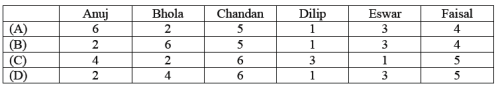*Answer can only contain numeric values
MA Mathematics - 2014 GATE Paper (Practice Test) - Question 8

The smallest angle of a triangle is equal to two thirds of the smallest angle of a quadrilateral. The
ratio between the angles of the quadrilateral is 3:4:5:6. The largest angle of the triangle is twice its
smallest angle. What is the sum, in degrees, of the second largest angle of the triangle and the
largest angle of the quadrilateral?

(Important : you should answer only the numeric value)

MA Mathematics - 2014 GATE Paper (Practice Test) - Question 9

One percent of the people of country X are taller than 6 ft. Two percent of the people of country Y
are taller than 6 ft. There are thrice as many people in country X as in country Y. Taking both
countries together, what is the percentage of people taller than 6 ft?

MA Mathematics - 2014 GATE Paper (Practice Test) - Question 10

The monthly rainfall chart based on 50 years of rainfall in Agra is shown in the following figure.
Which of the following are true? (k percentile is the value such that k percent of the data fall below
that value)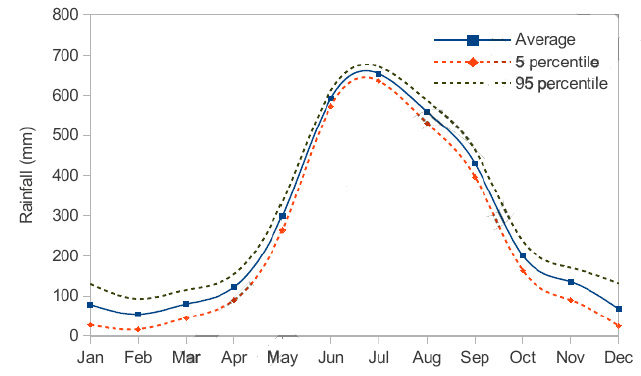(i) On average, it rains more in July than in December
(ii) Every year, the amount of rainfall in August is more than that in January
(iii) July rainfall can be estimated with better confidence than February rainfall
(iv) In August, there is at least 500 mm of rainfall

MA Mathematics - 2014 GATE Paper (Practice Test) - Question 11

Q. 1 – Q. 25 carry one mark each.

Q.

The function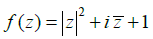is differentiable at

*Answer can only contain numeric values
MA Mathematics - 2014 GATE Paper (Practice Test) - Question 12

The radius of convergence of the power series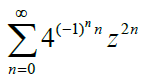is _____________

MA Mathematics - 2014 GATE Paper (Practice Test) - Question 13

Let   E1 and E2 be two non empty subsets of a normed linear space X and let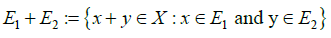Then which of the following statements is FALSE:

*Answer can only contain numeric values
MA Mathematics - 2014 GATE Paper (Practice Test) - Question 14

Let y(x) be the solution to the initial value problem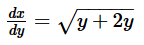subject to y(1.2) 2. Using the Euler method with the step size h = 0.05, the approximate value of ??(1.3), correct to two
decimal places, is _____________________

*Answer can only contain numeric values
MA Mathematics - 2014 GATE Paper (Practice Test) - Question 15

Let α ∈ R. If αx is the polynomial which interpolates the function f (x) = sinπ x on [−1,1]at all the zeroes of the polynomial 4x3 − 3x , then α is ___________

*Answer can only contain numeric values
MA Mathematics - 2014 GATE Paper (Practice Test) - Question 16

If u(x,t) is the D’Alembert’s solution to the wave equation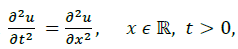with
the condition u(x,0) = 0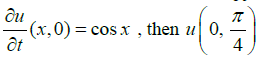is _________________

MA Mathematics - 2014 GATE Paper (Practice Test) - Question 17

The solution to the integral equation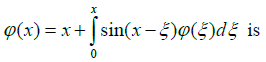MA Mathematics - 2014 GATE Paper (Practice Test) - Question 18

The general solution to the ordinary differential equation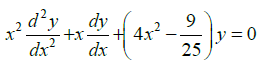in
terms of Bessel’s functions, ??v(x), is

MA Mathematics - 2014 GATE Paper (Practice Test) - Question 19

The inverse Laplace transform of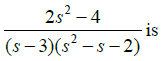MA Mathematics - 2014 GATE Paper (Practice Test) - Question 20

If  X1 , X2 is a random sample of size 2 from an (0,1) population, then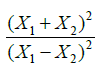follows

MA Mathematics - 2014 GATE Paper (Practice Test) - Question 21

Let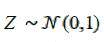be a random variable. Then the value of E[max{Z,0}] is

*Answer can only contain numeric values
MA Mathematics - 2014 GATE Paper (Practice Test) - Question 22

The number of non-isomorphic groups of order 10 is ___________

MA Mathematics - 2014 GATE Paper (Practice Test) - Question 23

Let a,b,c,d be real numbers with a < c < d < b. Consider the ring C[a,b] with pointwise
addition and multiplication. If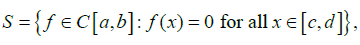then

MA Mathematics - 2014 GATE Paper (Practice Test) - Question 24

Let ?? be a ring. If R[x]is a principal ideal domain, then R is necessarily a

MA Mathematics - 2014 GATE Paper (Practice Test) - Question 25

Consider the group homomorphism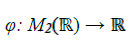given by φ ( A) = trace(A) . The kernel of φ
is isomorphic to which of the following groups?

MA Mathematics - 2014 GATE Paper (Practice Test) - Question 26

Let X be a set with at least two elements. Let τ and τ′ be two topologies on X such that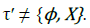Which of the following conditions is necessary for the identity function id :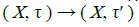to be
continuous?

*Answer can only contain numeric values
MA Mathematics - 2014 GATE Paper (Practice Test) - Question 27

Let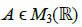be such that det(A− I ) = 0 , where I denotes the 3×3 identity matrix. If the
trace(A) =13 and det(A) = 32, then the sum of squares of the eigenvalues of A is ______

MA Mathematics - 2014 GATE Paper (Practice Test) - Question 28

Let V denote the vector space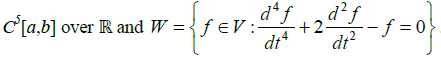. Then

*Answer can only contain numeric values
MA Mathematics - 2014 GATE Paper (Practice Test) - Question 29

Let V be a real inner product space of dimension 10 . Let x, y∈V be non-zero vectors such that
= 0 . Then the dimension of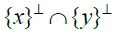is _________________

*Answer can only contain numeric values
MA Mathematics - 2014 GATE Paper (Practice Test) - Question 30

Consider the following linear programming problem:
Minimize x1 + x2
Subject to:
2x1 + x2 ≥ 8
2x1 + 5x2 ≥ 10
x1, x2 ≥ 0
The optimal value to this problem is _________________________

*Answer can only contain numeric values
MA Mathematics - 2014 GATE Paper (Practice Test) - Question 31

Let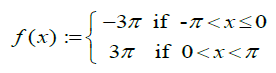be a periodic function of period 2π. The coefficient of sin 3x in the Fourier series expansion of f(x) on the interval [−π, π] is ________________________

MA Mathematics - 2014 GATE Paper (Practice Test) - Question 32

For the sequence of functions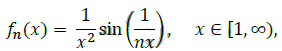consider the following quantities expressed in terms of Lebesgue integrals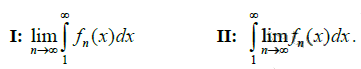Which of the following is TRUE?

MA Mathematics - 2014 GATE Paper (Practice Test) - Question 33

Which of the following statements about the spaces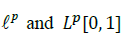is TRUE ?

MA Mathematics - 2014 GATE Paper (Practice Test) - Question 34

The maximum modulus of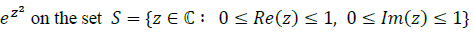is

MA Mathematics - 2014 GATE Paper (Practice Test) - Question 35

Let d1, d2 and d3 be metrics on a set X with at least two elements. Which of the following is NOT
a metric on X?

MA Mathematics - 2014 GATE Paper (Practice Test) - Question 36

Q. 26 – Q. 55 carry two marks each

Q.

Let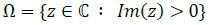and let ?? be a smooth curve lying in Ω with initial point −1 + 2??
and final point 1 + 2??. The value of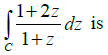*Answer can only contain numeric values
MA Mathematics - 2014 GATE Paper (Practice Test) - Question 37

If ? ? C with a <1, then the value of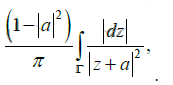where Γ is the simple closed curve |??| = 1 taken with the positive orientation, is _________

*Answer can only contain numeric values
MA Mathematics - 2014 GATE Paper (Practice Test) - Question 38

Consider C[−1,1] equipped with the supremum norm given by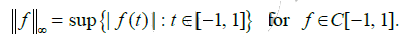Define a linear functional T on C[−1,1]by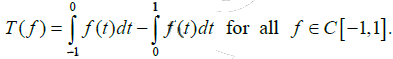Then the value of T is _______

MA Mathematics - 2014 GATE Paper (Practice Test) - Question 39

Consider the vector space C[0,1] over R. Consider the following statements:
P: If the set {  tf1, t2f2, t3f3} is linearly independent, then the set { f1,f2,f3} is linearly
independent, where f1,f2,f3  ∈C [0,1] and tn represents the polynomial function t→ tn, n ? N

Q: If F: C[0,1] → R is given by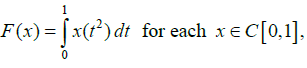then F is a linear map

Which of the above statements hold TRUE?

*Answer can only contain numeric values
MA Mathematics - 2014 GATE Paper (Practice Test) - Question 40

Using the Newton-Raphson method with the initial guess x(0) = 6, the approximate value of the
real root of x log10 x = 4.77 , after the second iteration, is ____________________

*Answer can only contain numeric values
MA Mathematics - 2014 GATE Paper (Practice Test) - Question 41

Let the following discrete data be obtained from a curve ?? = ??(??):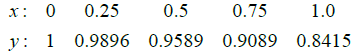Let S be the solid of revolution obtained by rotating the above curve about the ??-axis between x = 0 and ?? = 1 and let V denote its volume. The approximate value of V, obtained using
Simpson’s 1/3 rule, is ______________

MA Mathematics - 2014 GATE Paper (Practice Test) - Question 42

The integral surface of the first order partial differential equation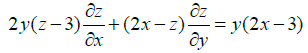passing through the curve x2 + y2 = 2x, z = 0 is

*Answer can only contain numeric values
MA Mathematics - 2014 GATE Paper (Practice Test) - Question 43

The boundary value problem,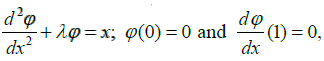? is converted into the
integral equation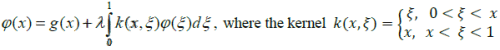Then g(2/3) is

MA Mathematics - 2014 GATE Paper (Practice Test) - Question 44

If ??1(??) = ?? is a solution to the differential equation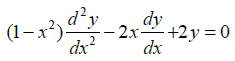then its
general solution is

MA Mathematics - 2014 GATE Paper (Practice Test) - Question 45

The solution to the initial value problem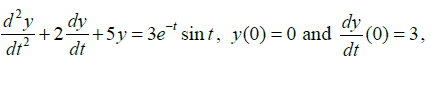is

*Answer can only contain numeric values
MA Mathematics - 2014 GATE Paper (Practice Test) - Question 46

The time to failure, in months, of light bulbs manufactured at two plants A and B obey the exponential distribution with means 6 and 2 months respectively. Plant B produces four times as many bulbs as plant A does. Bulbs from these plants are indistinguishable. They are mixed and sold together. Given that a bulb purchased at random is working after 12 months, the probability that it was manufactured at plant A is _____

*Answer can only contain numeric values
MA Mathematics - 2014 GATE Paper (Practice Test) - Question 47

Let X , Y be continuous random variables with joint density function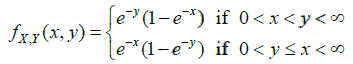The value of E[X +Y ]is ____________________

MA Mathematics - 2014 GATE Paper (Practice Test) - Question 48

Let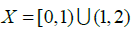be the subspace of R, where R is equipped with the usual topology. Which
of the following is FALSE?

MA Mathematics - 2014 GATE Paper (Practice Test) - Question 49

Let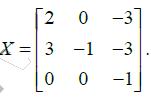A matrix P such that P−1XP is a diagonal matrix, is

MA Mathematics - 2014 GATE Paper (Practice Test) - Question 50

Using the Gauss-Seidel iteration method with the initial guess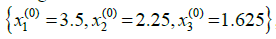,the second approximation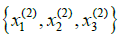for the solution to the system of equations

2x1-x2=7

-x1+2x2-x3=1

-x2+2x3=1

is

*Answer can only contain numeric values
MA Mathematics - 2014 GATE Paper (Practice Test) - Question 51

The fourth order Runge-Kutta method given by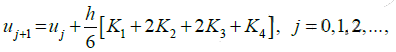is used to solve the initial value problem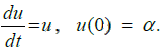If u(1) = 1 is obtained by taking the step size h = 1, then the value of 4 K is ______________

MA Mathematics - 2014 GATE Paper (Practice Test) - Question 52

A particle P of mass m moves along the cycloid x = (θ − sin θ) and ?? = (1 + cos θ),
0 ≤ θ ≤ 2??. Let g denote the acceleration due to gravity. Neglecting the frictional force, the
Lagrangian associated with the motion of the particle P is:

*Answer can only contain numeric values
MA Mathematics - 2014 GATE Paper (Practice Test) - Question 53

Suppose that ?? is a population random variable with probability density function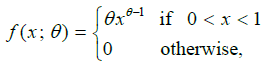where θ is a parameter. In order to test the null hypothesis H0: θ = 2, against the alternative
hypothesis H1: θ = 3, the following test is used: Reject the null hypothesis if X1 ≥ 1/2 and accept otherwise, where  X1 is a random sample of size 1 drawn from the above population. Then the power of the test is _____

MA Mathematics - 2014 GATE Paper (Practice Test) - Question 54

Suppose that x1, x2,…, xn is a random sample of size n drawn from a population with probability
density function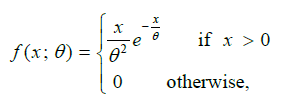where θ is a parameter such that θ > 0. The maximum likelihood estimator of θ is

*Answer can only contain numeric values
MA Mathematics - 2014 GATE Paper (Practice Test) - Question 55

Let F be a vector field defined on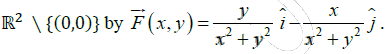Let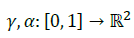be defined by ??(t) = (8 cos 2πt, 17 sin 2πt) and ??(t) = (26 cos 2πt, −10 sin 2πt).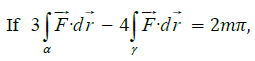then m is _________________________

*Answer can only contain numeric values
MA Mathematics - 2014 GATE Paper (Practice Test) - Question 56

Let g: R3 → R3 be defined by g(x, y, z) = (3y + 4z, 2x − 3z, x + 3y) and let

S = {(x, y, z) ∈ R3 : 0 ≤ x ≤ 1, 0 ≤ y ≤ 1, 0 ≤ z ≤ 1 }. If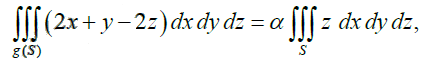then α is _____________________

*Answer can only contain numeric values
MA Mathematics - 2014 GATE Paper (Practice Test) - Question 57

Let T1, T2 : R5 → R3 be linear transformations such that rank(T1) = 3 and nullity(T2) = 3. Let
T3 : R3 → R3 be a linear transformation such that T3 ° T, = T2. Then rank(T3) is __________

*Answer can only contain numeric values
MA Mathematics - 2014 GATE Paper (Practice Test) - Question 58

Let F3 be the field of 3 elements and let F3 × F3 be the vector space over F3. The number of
distinct linearly dependent sets of the form {u, v}, where u, v ∈ F3 × F3 {(0,0)} and u ≠ v
is _____________

*Answer can only contain numeric values
MA Mathematics - 2014 GATE Paper (Practice Test) - Question 59

Let F125 be the field of 125 elements. The number of non-zero elements α ∈ F125 such that
α5 = α is _______________________

*Answer can only contain numeric values
MA Mathematics - 2014 GATE Paper (Practice Test) - Question 60

The value of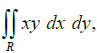where R is the region in the first quadrant bounded by the curves
y = x2, y + x = 2 and x = 0 is ______________

*Answer can only contain numeric values
MA Mathematics - 2014 GATE Paper (Practice Test) - Question 61

Consider the heat equation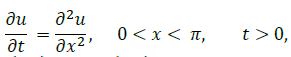with the boundary conditions u(0, t) = 0, u(π, t) = 0 for t > 0, and the initial condition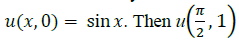is ___________________

MA Mathematics - 2014 GATE Paper (Practice Test) - Question 62

Consider the partial order in R2 given by the relation (x1, y1) < (x2, y2) EITHER if x1 < x2 OR
if x1 = x2 and y1 < y2. Then in the order topology on R2 defined by the above order

MA Mathematics - 2014 GATE Paper (Practice Test) - Question 63

Consider the following linear programming problem:
Minimize: x1 + x2 + 2x3
Subject to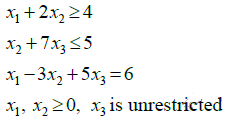The dual to this problem is:
Maximize: 4y1 +5y2 + 6y3
Subject to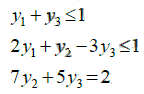and further subject to:

MA Mathematics - 2014 GATE Paper (Practice Test) - Question 64

Let X = C1[0,1]. For each f ∈ X , define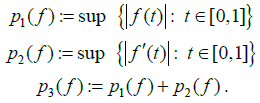Which of the following statements is TRUE?

MA Mathematics - 2014 GATE Paper (Practice Test) - Question 65

If the power series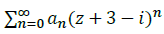converges at 5i and diverges at −3i, then the power series

## GATE Past Year Papers for Practice (All Branches)

380 docs|127 tests
 Use Code STAYHOME200 and get INR 200 additional OFF Use Coupon Code
Information about MA Mathematics - 2014 GATE Paper (Practice Test) Page
In this test you can find the Exam questions for MA Mathematics - 2014 GATE Paper (Practice Test) solved & explained in the simplest way possible. Besides giving Questions and answers for MA Mathematics - 2014 GATE Paper (Practice Test), EduRev gives you an ample number of Online tests for practice

## GATE Past Year Papers for Practice (All Branches)

380 docs|127 tests